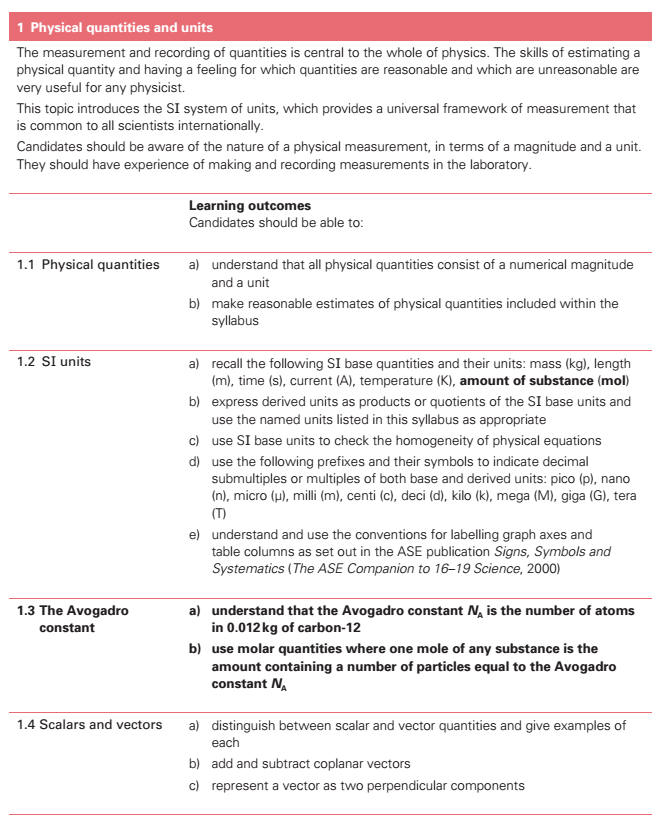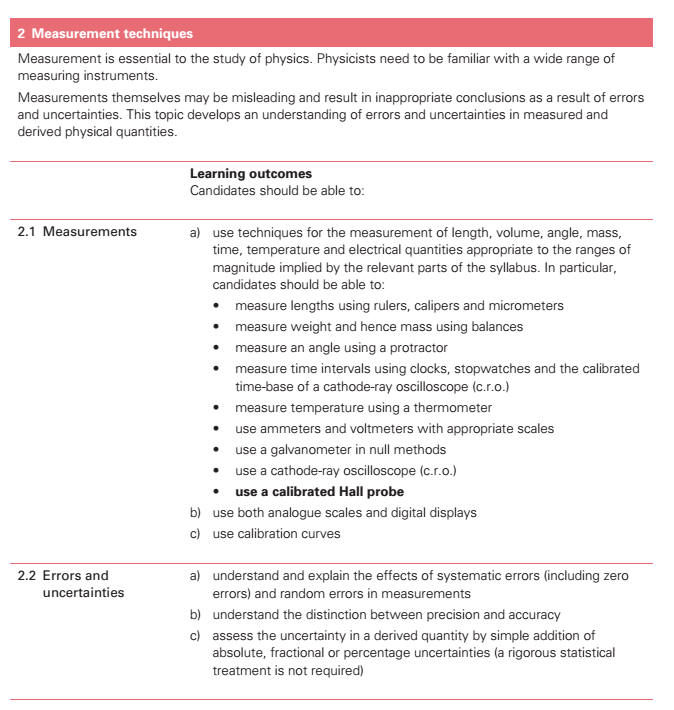1. Physical Quantities and Units[] (a) show an understanding that all physical quantities consist of a numerical magnitude and a unit. (b) recall the following base quantities and their units: mass (kg), length (m), time (s), current (A), temperature[] (K), amount of substance (mol). (c) express derived units[] as products or quotients of the base units[] and use the named units[] listed in this syllabus as appropriate. (d) use base units[] to check the homogeneity of physical equations. (e) show an understanding and use the conventions for labelling graph axes and table columns (f) use the following prefixes and their symbols to indicate decimal sub-multiples or multiples of both base and derived units: pico (p), nano (n), micro (µ), milli (m), centi (c), deci (d), kilo (k), mega (M), giga (G), tera (T). (g) make reasonable estimates of physical quantities included within the syllabus. (h) show an understanding of the significance of the Avogadro constant as the number of atoms in 0.012 kg of Carbon-12. (i) use molar quantities where one mole[] of any substance is the amount containing a number of particles equal to the Avogadro constant. (j) distinguish scalar and vector quantities and give examples of each. (k) add and subtract coplanar vectors[]. (l) represent a vector as two perpendicular components. 2 . Measurement Techniques (a) use techniques for the measurement of length, volume, angle, mass, time, temperature[] and electrical quantities appropriate to the ranges of magnitude implied by the relevant parts of the syllabus. In particular, candidates should be able to: (1) measure lengths using a ruler, vernier scale and micrometer[]. (2) measure weight and hence mass using spring and lever balances. (3) measure an angle using a protractor. (4) measure time intervals using clocks, stopwatches and the calibrated time-base of a cathode-ray oscilloscope (cro). (5) measure temperature[] using a thermometer as a sensor. (6) use ammeters and voltmeters with appropriate scales. (7) use a galvanometer in null methods. (8) use a cathode-ray oscilloscope cro[]. (9) use a calibrated Hall probe. * (b) use both analogue scales and digital displays. * (c) use calibration[] curves. (d) show an understanding of the distinction between systematic errors (including zero errors) and random errors. (e) show an understanding of the distinction between precision and accuracy. * (f) assess the uncertainty[] in a derived quantity by simple addition of actual, fractional or percentage uncertainties (a rigorous statistical treatment is not required).